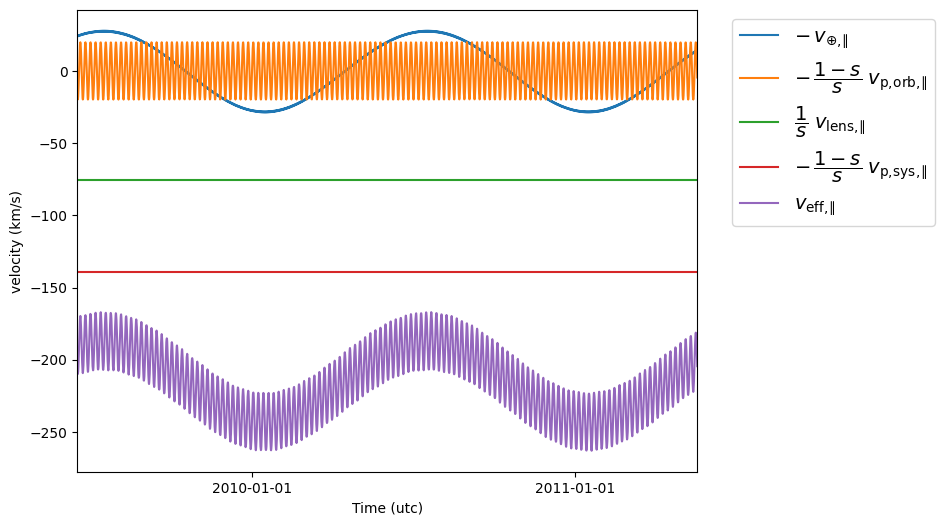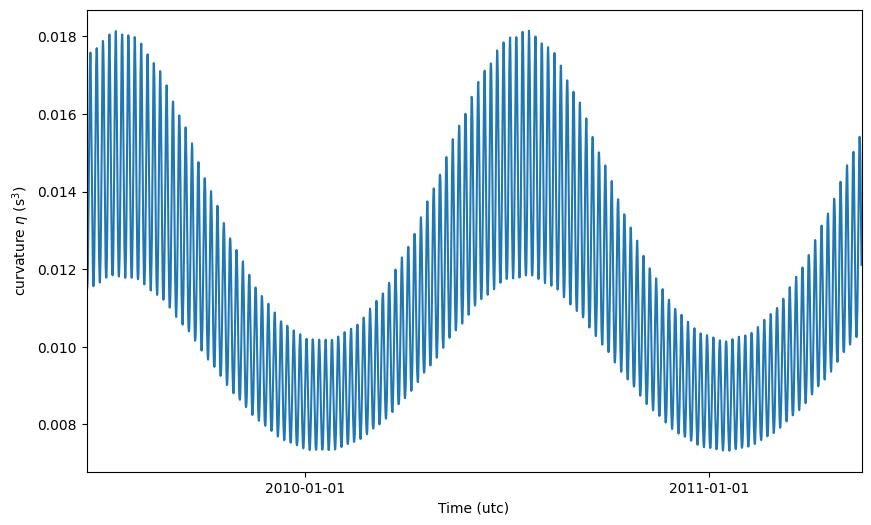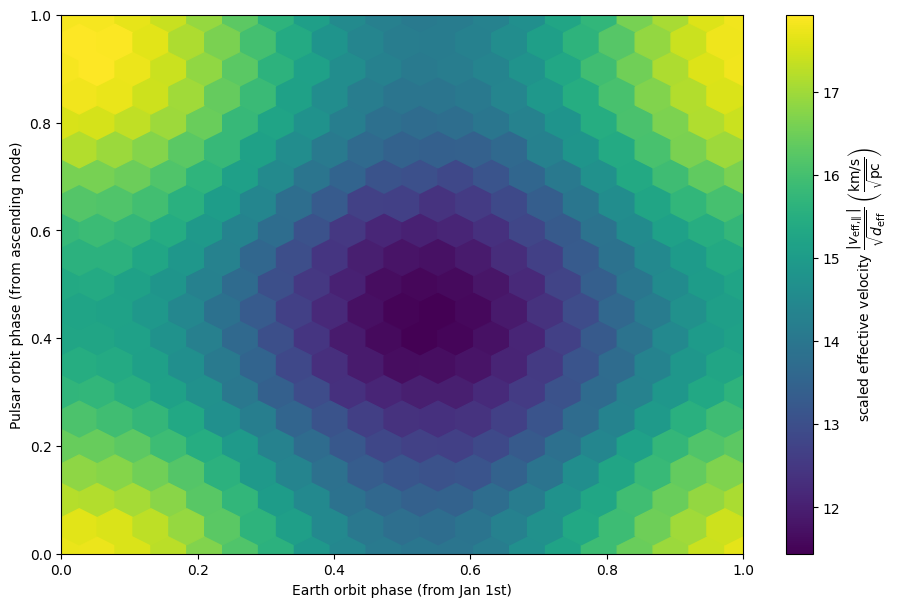# Generating synthetic scintillation velocities¶

This tutorial describes how to generate synthetic scintillation velocities for a pulsar in a circular orbit and a single one-dimensional screen, both with known parameters.

For a derivation of the equations seen here, refer to the scintillation velocities background. Further explanations can be found in Marten’s scintillometry page and Daniel Baker’s “Orbital Parameters and Distances” document. As in that document, the practical example here uses the parameter values for the pulsar PSR J0437–4715 as derived by Reardon et al. (2020).

The combined codeblocks in this tutorial can be downloaded as a Python script and as a Jupyter notebook:

Python script:

gen_velocities.py

Jupyter notebook:

gen_velocities.ipynb

## Preliminaries¶

Imports.

import numpy as np
import matplotlib.pyplot as plt

from astropy import units as u
from astropy import constants as const

from astropy.time import Time
from astropy.coordinates import SkyCoord, SkyOffsetFrame, EarthLocation

from astropy.visualization import quantity_support, time_support


Set up support for plotting Astropy’s Quantity and Time objects, and make sure that the output of plotting commands is displayed inline (i.e., directly below the code cell that produced it).

quantity_support()
time_support(format='iso')

%matplotlib inline


## Initializing parameters¶

Set the parameters of the pulsar system:

Parameter

Symbol

Remarks

Coordinates of the pulsar system

right ascension

$$\alpha_\mathrm{p}$$

declination

$$\delta_\mathrm{p}$$

proper motion in right ascension

$$\mu_\mathrm{p,sys,\alpha\ast}$$

including the $$\cos(\delta_\mathrm{p})$$ term

proper motion in declination

$$\mu_\mathrm{p,sys,\delta}$$

Orbital elements of the pulsar binary

binary period

$$P_\mathrm{orb,p}$$

projected semi-major axis

$$a_\mathrm{p} \sin( i_\mathrm{p} )$$

orbital inclination

$$i_\mathrm{p}$$

$$0^\circ \leq i_\mathrm{p} < 180^\circ$$, with $$i_\mathrm{p} = 90^\circ$$ for an edge-on orbit; for $$i_\mathrm{p} < 90^\circ$$, the binary rotates counterclockwise on the sky (from north through east), for $$i_\mathrm{p} \geq 90^\circ$$ it rotates clockwise

longitude of ascending node

$$\Omega_\mathrm{p}$$

measured from the celestial north through east; $$0^\circ \leq \Omega_\mathrm{p} < 360^\circ$$

time of ascending node

$$t_\mathrm{asc,p}$$

Further parameters

distance to the pulsar system

$$d_\mathrm{p}$$

distance to the screen

$$d_\mathrm{s}$$

from Earth

position angle of the lensed images

$$\xi$$

measured from the celestial north, through east, to the line of images formed by the lens; $$0^\circ \leq \xi < 180^\circ$$

velocity of the lens

$$v_\mathrm{lens,\parallel}$$

component along the screen direction

p_orb_p = 5.7410459 * u.day
asini_p = 3.3667144 * const.c * u.s
i_p = 137.56 * u.deg
omega_p = 207. * u.deg
t_asc_p = Time(54501.4671, format='mjd', scale='tdb')

d_p = 156.79 * u.pc
d_s = 90.6 * u.pc
xi = 134.6 * u.deg
v_lens = -31.9 * u.km / u.s


The coordinates should be placed directly in a SkyCoord object, that includes the pulsar system’s position on the sky, its distance, and its proper motion.

psr_coord = SkyCoord('04h37m15.99744s -47d15m09.7170s',
distance=d_p,
pm_ra_cosdec=121.4385 * u.mas / u.yr,
pm_dec=-71.4754 * u.mas / u.yr)


Calculate some derived quantities:

Parameter

Equation

$K_\mathrm{p} = \frac{ 2 \pi a_\mathrm{p} \sin( i_\mathrm{p} ) } { P_\mathrm{orb,p} }$

fractional distance to the screen (from the pulsar)

$s = 1 - \frac{ d_\mathrm{s} }{ d_\mathrm{p} }$

effective distance

$d_\mathrm{eff} = \frac{ 1 - s }{ s } d_\mathrm{p}$

angle from the pulsar’s ascending node to the line of lensed images

$\Delta\Omega_\mathrm{p} = \xi - \Omega_\mathrm{p}$
k_p = 2.*np.pi * asini_p / p_orb_p

s = 1 - d_s / d_p
d_eff = d_p * d_s / (d_p - d_s)

delta_omega_p = xi - omega_p


Define a grid of observing times $$t$$ for which you want to calculate velocities using a Time object.

t_mjd = np.arange(55000., 55700., 0.25)
t = Time(t_mjd, format='mjd', scale='utc')


## The lens frame¶

Make a SkyOffsetFrame centered on the pulsar system, rotated to the one-dimensional lens.

lens_frame = SkyOffsetFrame(origin=psr_coord, rotation=xi)


On its own, SkyOffsetFrame(origin=psr_coord) creates a spherical frame with its primary direction pointing along the line of sight, latitude in the direction of Dec, and longitude in the direction of RA. By passing the argument rotation=xi, the longitude and latitude dimensions rotate so longitude is perpedicular to the lens and latitude parallel to the lens. When converting positions or velocities in this frame to cartesian representation, the x-axis will point along the line of sight, the y-axis perpendicular to the screen, and the z-axis parallel to the screen (in the direction of its motion). Hence, we need to compute the cartesian z-component of velocities in lens_frame.

## Calculating effective velocities¶

There are several components of the effective velocity that can be computed separately:

Velocity component

Symbol

Earth’s velocity as a function of time

$$v_{\oplus,\parallel}( t )$$

pulsar’s orbital velocity as a function of time

$$v_\mathrm{p,orb,\parallel}( t )$$

pulsar systemic velocity (corresponding to the proper motion)

$$v_\mathrm{p,sys,\parallel}$$

velocity of the lens (known in this example)

$$v_\mathrm{lens,\parallel}$$

All these refer to the component of the velocity along the line of images formed by the lens.

### Earth’s velocity¶

To obtain Earth’s velocity in the lens frame, first generate a location on Earth’s surface using the EarthLocation class (in this case the location of the Parkes radio telescope). This class has the get_gcrs() method, which returns positions (with respect to the centre of the Earth) as a function of time. These are transformed into the lens frame using the transform_to() method. Velocities can then be extracted using the velocity attribute, and finally d_z isolates the z-component of the velocity (in the direction of the screen).

earth_loc = EarthLocation('148°15′47″E', '32°59′52″S')

v_earth = earth_loc.get_gcrs(t).transform_to(lens_frame).velocity.d_z


### Pulsar’s orbital velocity¶

Compute the pulsar’s orbital velocity projected onto the screen

$v_\mathrm{p,orb,\parallel} = - \frac{ K_\mathrm{p} }{ \sin( i_\mathrm{p} ) } \left[ \cos( \Delta\Omega_\mathrm{p} ) \sin( \phi_\mathrm{p} ) - \sin( \Delta\Omega_\mathrm{p} ) \cos( i_\mathrm{p} ) \cos( \phi_\mathrm{p} ) \right].$

Here, $$\phi_\mathrm{p}( t )$$ is the phase of pulsar orbit as measured from its ascending node.

ph_p = ((t - t_asc_p) / p_orb_p).to(u.dimensionless_unscaled) * u.cycle

v_p_orb = (-k_p / np.sin(i_p)
* (np.cos(delta_omega_p) * np.sin(ph_p)
- np.sin(delta_omega_p) * np.cos(i_p) * np.cos(ph_p)))


### Pulsar systemic velocity¶

The pulsar systemic velocity projected onto the screen is given by

$v_\mathrm{p,sys,\parallel} = d_\mathrm{p} \left[ \mu_\mathrm{p,sys,\alpha\ast} \sin( \xi ) + \mu_\mathrm{p,sys,\delta} \cos( \xi ) \right].$

This can be computed manually, but it can also be retrieved from the SkyCoord of the pulsar system (which contains the system’s proper motion) by transforming it to lens_frame.

v_p_sys = psr_coord.transform_to(lens_frame).velocity.d_z


### Effective velocity¶

Combine the velocities of the pulsar, Earth, and the lens into the effective velocity

$v_\mathrm{eff,\parallel} = \frac{1}{s} v_\mathrm{lens,\parallel} - \frac{1 - s}{s} \left( v_\mathrm{p,sys,\parallel} + v_\mathrm{p,orb,\parallel} \right) - v_{\oplus,\parallel}$
v_eff = 1. / s * v_lens - (1. - s) / s * (v_p_sys + v_p_orb) - v_earth


Have a look at the contribution of each of the terms to the effective velocity.

plt.figure(figsize=(8., 6.))

plt.plot(t, - v_earth)
plt.plot(t, - ((1. - s) / s) * v_p_orb)
plt.plot(t[::len(t)-1], 1. / s * v_lens * [1., 1.])
plt.plot(t[::len(t)-1], - ((1. - s) / s) * v_p_sys * [1., 1.])
plt.plot(t, v_eff)
plt.legend([r'$- \, v_{\oplus,\!\!\parallel}$',
r'$- \, \dfrac{ 1 - s }{ s } \; v_\mathrm{p,\!orb,\!\!\parallel}$',
r'$\dfrac{ 1 }{ s } \; v_\mathrm{lens,\!\!\parallel}$',
r'$- \, \dfrac{ 1 - s }{ s } \; v_\mathrm{p,\!sys,\!\!\parallel}$',
r'$v_\mathrm{eff,\!\!\parallel}$'],
bbox_to_anchor=(1.04, 1.), loc='upper left', fontsize=14)
plt.xlim(t, t[-1])
plt.ylabel(r'velocity (km/s)')

plt.show()## Curvature and scaled effective velocity¶

The curvature $$\eta$$ can be computed from the effective velocity according to

$\eta = \frac{ \lambda^2 d_\mathrm{eff} }{ 2 c v_\mathrm{eff,\parallel}^2 },$

where $$\lambda$$ is the observing wavelength and $$c$$ is the speed of light.

lambda_obs = (1400. * u.MHz).to(u.m, equivalencies=u.spectral())

eta = lambda_obs**2 * d_eff / (2. * const.c * v_eff**2)


Have a look at the curvature at a function of time.

plt.figure(figsize=(10., 6.))

plt.plot(t, eta.to(u.s**3))
plt.xlim(t, t[-1])
plt.ylabel(r'curvature $\eta$ (s$^3$)')

plt.show()Since $$v_\mathrm{eff}$$ can be arbitrarily close to zero (letting $$\eta$$ blow up), curvature has a strongly non-uniform prior probability distribution (as can be seen from the modulation in amplitude in the figure above). For this reason, it is sometimes better to fit for the curvature of the secondary spectrum parabola in a space of “scaled effective velocity”

$\frac{ \lambda }{ \sqrt{ 2 \eta c } } = \frac{ \left| v_\mathrm{eff,\parallel} \right| } { \sqrt{ d_\mathrm{eff} } }$
dveff = np.abs(v_eff) / np.sqrt(d_eff)


Plot this quantity as function of time.

plt.figure(figsize=(10., 6.))

plt.plot(t, dveff)
plt.xlim(t, t[-1])
dveff_lbl = (r'scaled effective velocity '
r'$\dfrac{ | v_\mathrm{eff,\!\!\parallel} | }' r'{ \sqrt{ d_\mathrm{eff} } }$ '
r'$\left( \dfrac{\mathrm{km/s}}{\sqrt{\mathrm{pc}}} \right)$')
plt.ylabel(dveff_lbl)

plt.show()To visualize the modulation in scintillation velocity caused by both the pulsar’s orbital motion and that of the Earth, we can make a 2D phase fold of the data.

plt.figure(figsize=(11., 7.))

plt.hexbin(t.jyear % 1., ph_p.value % 1., C=dveff.value,
reduce_C_function=np.median, gridsize=19)
plt.xlim(0., 1.)
plt.ylim(0., 1.)
plt.xlabel('Earth orbit phase (from Jan 1st)')
plt.ylabel('Pulsar orbit phase (from ascending node)')
cbar = plt.colorbar()
cbar.set_label(dveff_lbl)

plt.show()## Generate noisy synthetic observations¶

We now want to generate a set of noisy scaled effective velocities, to use in the next tutorial, in which we will fit a model to these fake observations.

To start, we create a set of irregularly spaced observation times.

np.random.seed(654321)
nt = 2645
dt_mean = 16.425 * u.yr / nt
dt = np.random.random(nt) * 2. * dt_mean
t = Time(52618., format='mjd') + dt.cumsum()


Next, the time-dependent parts of the above calculations need to be repeated for the new times.

v_earth = earth_loc.get_gcrs(t).transform_to(lens_frame).velocity.d_z

ph_p = ((t - t_asc_p) / p_orb_p).to(u.dimensionless_unscaled) * u.cycle

v_p_orb = (-k_p / np.sin(i_p)
* (np.cos(delta_omega_p) * np.sin(ph_p)
- np.sin(delta_omega_p) * np.cos(i_p) * np.cos(ph_p)))

v_eff = 1. / s * v_lens - (1. - s) / s * (v_p_orb + v_p_sys) - v_earth

dveff = np.abs(v_eff) / np.sqrt(d_eff)


Now we add some noise to the scaled effective velocities.

dveff_err = (np.random.random(nt) * 0.05 + 0.05) * np.mean(dveff)
dveff_obs = dveff + dveff_err * np.random.normal(size=nt)


Finally, we use NumPy’s savez() to save the data as a set of (unitless) NumPy arrays.

# np.savez('data/fake-data-J0437.npz',
#          t_mjd=t.mjd,
#          dveff_obs=dveff_obs.value,
#          dveff_err=dveff_err.value)